Time: 3 Hours                                                                                                     Max. Marks: 100

NOTE: There are 9 Questions in all.

·      Question 1 is compulsory and carries 20 marks. Answer to Q. 1. must be written in the space provided for it in the answer book supplied and nowhere else.

·      Out of the remaining EIGHT Questions answer any FIVE Questions. Each question carries 16 marks.

·      Any required data not explicitly given, may be suitably assumed and stated.

Q.1       Choose the correct or best alternative in the following:                                         (2x10)

a.       The desired characteristics of a strain gauge are that it should have _________ and __________.

(A)  a resistance which is as low as possible, it should not have hysteresis effects in its response.

(B) A resistance which is as high as possible, it should have hysteresis effects is its response.

(C) a resistance which is as high as possible, it should not have hysteresis effects in its response.

(D) a resistance which is as low as practicable, it should have hysteresis effects in its response.

b.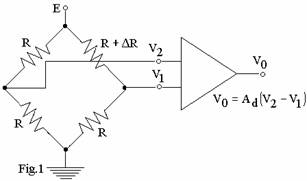Considering the differential bridge amplifier shown in Fig.1, if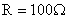,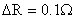and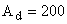, then,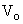will be nearly equal to ______ times the applied voltage E.

(A)    0.05.

(B)    0.0625.

(C)    0.005.

(D)    0.0125.

c.   The aperture time of the sample-and-hold circuit is __________

(A)    the time required for the switch to change state and the uncertainty in the time that this change of state occurs.

(B)    The length of time that the circuit can hold the charge without dropping more than a specified percentage of the initial value.

(C)    The time taken by the capacitor to charge upto half the value of the input voltage without any leakage.

(D)    The time taken by the capacitor to charge upto the value of the input voltage.

d.   Fixed standards which are kept for use in industrial laboratories are called _____________.

(A)    International standards.                (B)  Secondary standards.

(C)  Primary standards.                       (D)  Working standards.

e.   If in a Wien bridge circuit the measured frequency is 330 Hz and the resistors are each equal to 53.6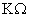, then each of the capacitors will be nearly equal to __________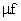.

(A)     0.007.                                          (B)  0.009.

(C)  0.011                                           (D)  0.102.

f.    If the temperature coefficient of resistance of copper is 0.0045 and its resistance at a temperature of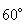C is 279.4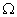then its resistance at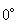C will be ______.

(A) 310                                               (B)  210

(C) 260                                               (D)  220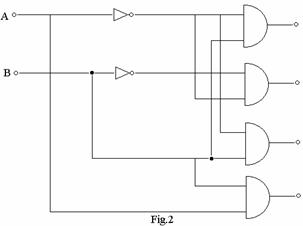g.   In the decoder circuit of Fig.2, an input of A = 0 and B = 1 will yield an output of __________.

(A)     1101

(B)     1011

(C)     1110

(D)    1010

h.   The degree of refinement with which a measured value is stated is called _____________

(A)    Resolution                                    (B) Stability

(C)  Precision                                      (D) Uncertainty

i.    One of the following equipment is not a passive transducer

(A) potentiometer element                    (B)  piezo resistive element

(C)  Thermistor                                    (D)  photovoltaic cells

j.    A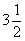-digit meter has a resolution of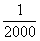th of the range and displays a maximum of __________.

(A)  1099                                            (B)  1999

(C)  1000                                            (D)  2000

Answer any FIVE Questions out of EIGHT Questions.

Each question carries 16 marks.

Q.2     a.   What is meant by word ‘Calibration’? Explain how and where it is done.             (6)

b.   Give the classification of ‘Standards’.   Describe in detail the setting up of any two of them.             (10)

Q.3           A bridge is balanced at 1000 Hz and has the following constants: AB,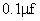pure capacitance; BC, 1000pure resistance; CD unknown; DA, R = 600in parallel with C = 0.05.  The terminals A and C are connected to the a.c. supply.  Find the R and C (or L) constants of the arm CD, assuming that it is a series circuit.                                        (16)

Q.4     a.   Explain the terms (i)  attenuator and (ii) probe as applied to a CRO.  Give the circuit of an active probe and describe its features.                            (8)

b.   Given the following data, compute the deflection sensitivity of a CRO:

Distance from the centre of the deflection plates to the screen (L)                    0.8m.

Effective length of the deflection plates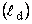0.05m.

Distance between the deflection plates (d)                                                    0.02m.

Accelerating voltage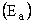1250V.

(8)

Q.5     a.   Distinguish between the pairs

(i)                  Primary sensors and transducers.

(ii)                Active and Passive transducers.                                                  (6)

b.   A resistance gauge with an unknown gauge factor is fastened to a steel member subjected to a stress of 1120 kg/cm2. The modulus of elasticity of steel is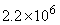kg/cm2 .  If the resistance of the unstrained gauge is     150 ohms and the change in resistance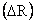is 0.3, calculate the gauge factor.              (10)

Q.6           Write notes on any TWO of the following:

(i)                  Different types of errors for time and frequency measurement.

(ii)                Obtaining hysteresis loop under a.c. operating conditions.

(iii)               Digital voltmeter.                                                                    (8+8)

Q.7     a.   A small AF voltage of 22 is superimposed on the RF test power and balance achieved.  If the RF test power is now turned off, an AF voltage of 34 is required to balance the bridge.  If the bridge arm has a resistance of 125, calculate the RF test power.                                                                  (7)

b.   In respect of radio receiver measurements, describe any THREE of the following:

FM receiver alignment, sweep alignment, non swept alignment, dual-sweep alignment.                    (9)

Q.8     a.   Explain the functions of the following parts of a spectrum analyser:

(i)   attenuator                                      (ii)  first IF filter

(iii) local oscillator                                (iv)  sweep voltage generator                   (8)

b.   Show the basic elements of a function generator by means of a block diagram.  Explain the purpose of a constant current source, multivibrator and resistance-diode shaping circuit in the same.        (8)

Q.9     a.   Explain the terms:

(iv)              aperture error and

(v)                quantization error occurring in an A/D converter.                        (4)

b.   For the D/A converter shown in Fig.4, compute the voltage

(i)                  with only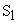closed and

(ii)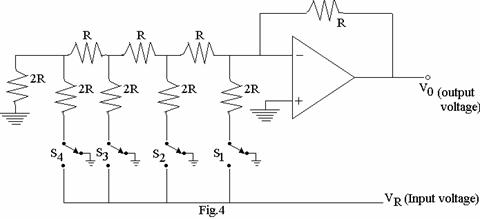with only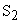closed.                                                                            (12)